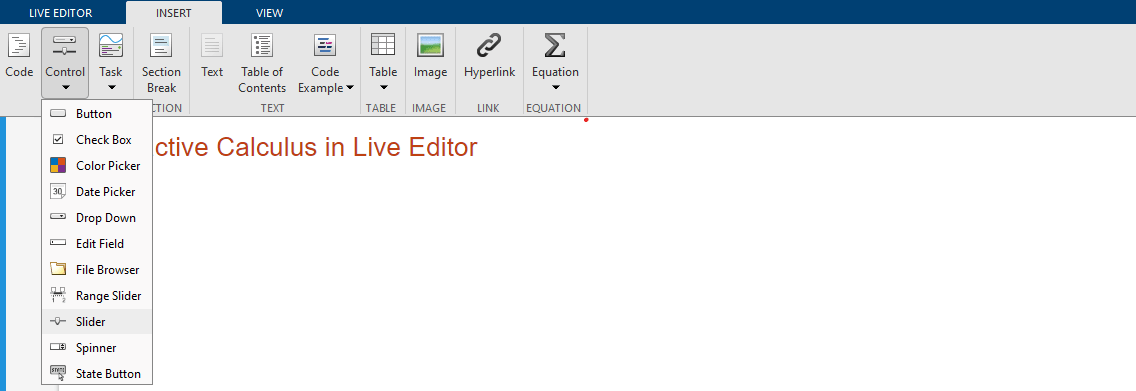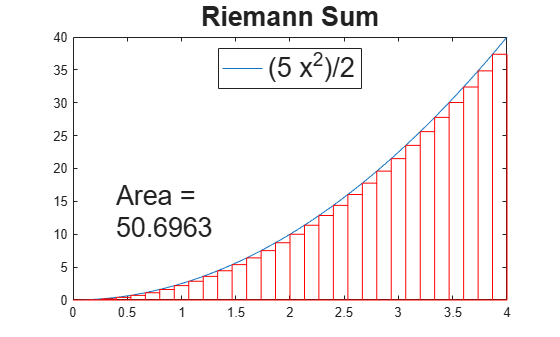Interactive Calculus in Live Editor

This example shows how you can add interactive controls to solve a calculus problem in a live script.

An interactive control can be used to change the values of variables in your live script. To add a numeric slider, go to the Insert tab, click the Control button, and select Numeric Slider. For more information, see Add Interactive Controls to a Live Script.Initialize Variables and Function

Evaluate the integral

${\int }_{0}^{{x}_{Max}}c{x}^{2}\phantom{\rule{0.2777777777777778em}{0ex}}dx$

using Riemann sum approximation.

A Riemann sum is a numerical approximation of the analytical integration by a finite sum of rectangular areas. Use the interactive slider bars to set the upper bound of the integral, the number of rectangles, and the constant factor of the function.

syms x;
xMax =4;
numRectangles =30;
c =2.5;
f(x) = c*x^2;
yMax = double(f(xMax));

Visualize the Area Under the Curve Using Riemann Sums

Plot the integrand f.

fplot(f);
xlim([0 xMax]); ylim([0 yMax]);
legend({}, 'Location', 'north', 'FontSize', 20);
title('Riemann Sum', 'FontSize', 20);

Calculate the rectangular areas that approximate the area under the curve of the integral. Plot the rectangles.

width = xMax/numRectangles;
sum = 0;
for i = 0:numRectangles-1
xval = i*width;
height = double(f(xval));
rectangle('Position', [xval 0 width height], 'EdgeColor', 'r');
sum = sum + width*height;
end
text(xMax/10, yMax/3, ['Area = ' num2str(sum)], 'FontSize', 20);Calculate the Integral Analytically

Calculate the integral analytically. Use vpa to numerically approximate the exact symbolic result to 32 significant digits.

fInt = int(f,0,xMax)
fInt =

$\frac{160}{3}$

vpa(fInt)
ans = $53.333333333333333333333333333333$

Symbolic Math Toolbox Documentation

Mathematical Modeling with Symbolic Math Toolbox

Get examples and videos